Yasushi Miki

## Using the Logistic Map to Generate Scratching SoundsNBCDFPDF

This article presents a mathematical model for generating annoying scratching sounds. Such sounds are generated by frictional motion and have been attributed to the chaotic nature of the frequency spectrum thereby produced. The proposed model is based on the logistic map and is modified to have the stick-slip property of a frictional vibration. The resulting sound is similar to that generated by scratching a chalkboard or glass plate with the fingernails.

### 1. Introduction

Recent research on annoying sounds generated by, for example, scratching on a chalkboard with fingernails has been presented from the viewpoint of psychoacoustics [1, 2]. We have previously examined those sounds from a physical standpoint. In the present article, however, we propose a mathematical model for generating a scratching sound. Such a sound can be generated from the stick-slip motion of a frictional system, and it is shown here that this mechanism is closely related to the logistic map. Since the logistic map provides only a periodic slip action, an additional property has to be introduced to produce a stick-slip action similar to bowing a stringed instrument.

### 2. Scratching-Sound Generator

#### 2.1. Frictional Motion and the Logistic Map

Particle motion in one dimension under the influence of periodic impulsive forces was described in . The logistic map shown below was derived from the strong-damping limit of the system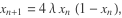(1)

where the parameter,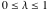, determines the range of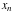.

#### 2.2. Chaotic Behavior of the Logistic Map

The logistic map is a classic example of chaotic behavior, and it is found to be strongly related to frictional vibration. The sound generated by the logistic map, with the parameterin equation (1) varying from 0.75 to 1, is considered in .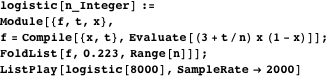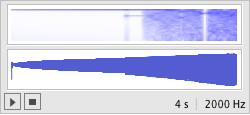Figure 1. Original sound. Sample rate: 2 kHz;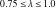.

This following slight modification of the program has a frequency spectrum similar to a scratching sound.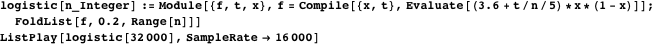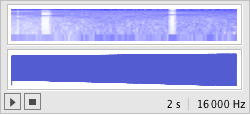Figure 2. High sample-rate sound. Sample rate: 16 kHz;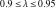.

One of the major modifications is that the sample rate is increased to 16 kHz. An annoying chilling reaction appears to coincide with the logistic system entering or leaving a chaotic phase.

#### 2.3. Representation of Stick-Slip Motion

The scratching model described above is a periodic slip model. Frictional motions in general are described as stick-slip phenomena. One of the most beautiful sounds to many people is that produced by bowing a violin or other stringed instrument. On the other hand, the sound produced by scratching a chalkboard with the fingernails is usually considered highly annoying. In both cases, however, the mechanism for producing sounds is the same, that is, the stick-slip phenomenon of a frictional system. This consists of a repetition of a stick stage and a slip stage. Figure 3 shows the sound for the actual stick-slip motion of a bowed string. Periodic motions are observed, with each period showing a steep rising motion followed by an interval of decrease. The former corresponds to the slip stage, the motion of short duration. The latter corresponds to the stick stage, where the string moves at a constant speed along with the bow and continues until the slip occurs.

To import the stick-slip property into the logistic model, we introduce the following rules:

(a) If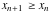, in equation (1), transition occurs immediately. This is the slip motion.

(b) If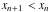, the value ofwithin (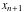,) decreases linearly at a constant speed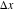. This is the stick motion, and the valuedetermines the speed of the bow.

Thus we obtain the following modified logistic map representing the stick-slip phenomenon.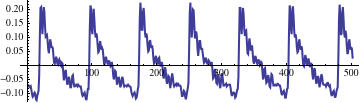Figure 3. Waveform of the bowed string. Sampling frequency: 50 kHz. Number of points: 500.

#### 2.4. The Scratching-Sound Generator

The flow chart of the new logistic model discussed above is shown in Figure 4. The left branch describes the stick stage and the right branch describes the slip stage. The velocity parametergives the bowing speed.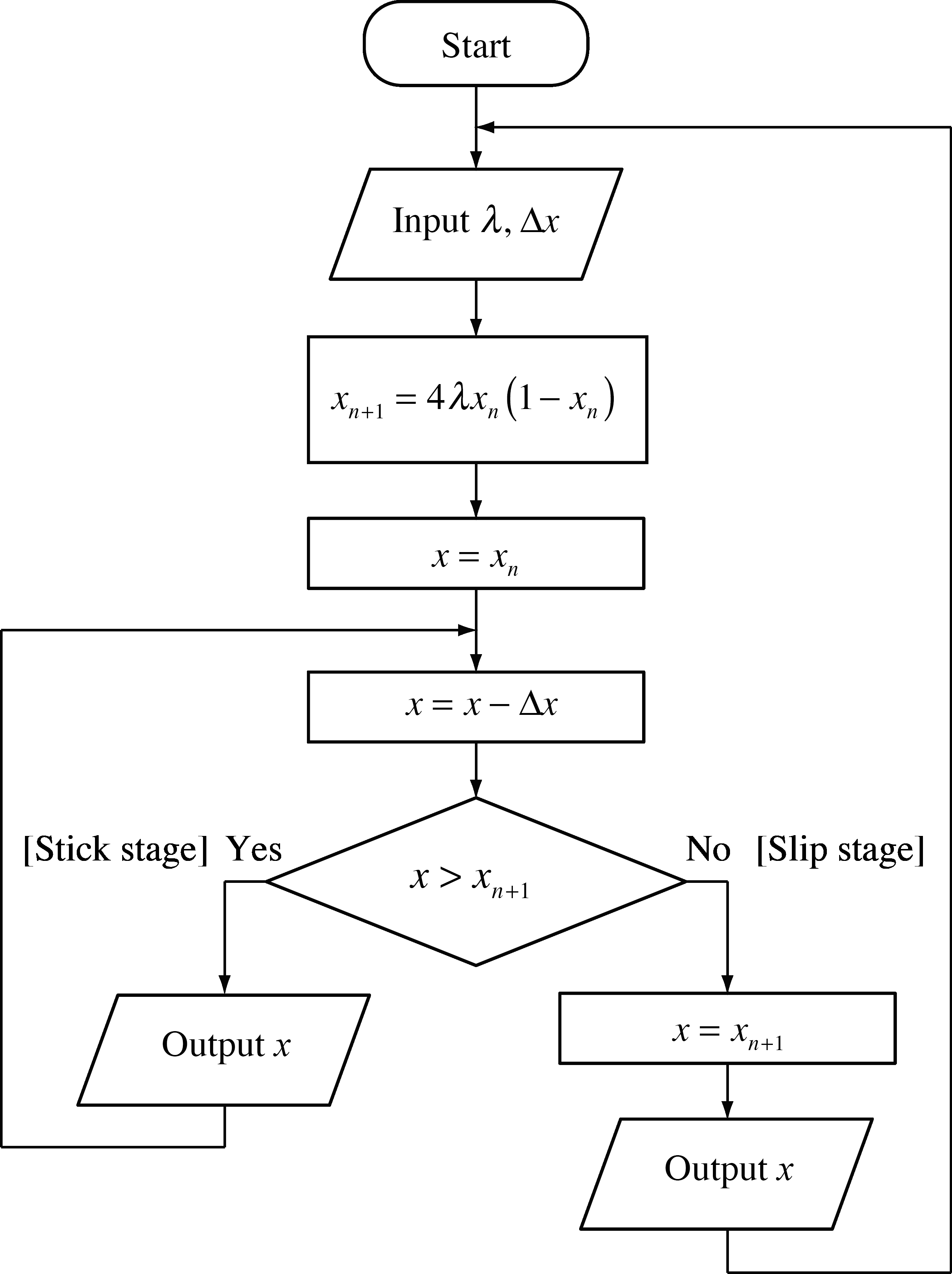Figure 4. Flow chart of the modified logistic model.

Here we show the behavior of the modified logistic model. The valuegives the height of the parabola and can be varied from 0.5 to 1, while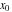is the initial value of.

First we define the logistic function f.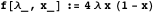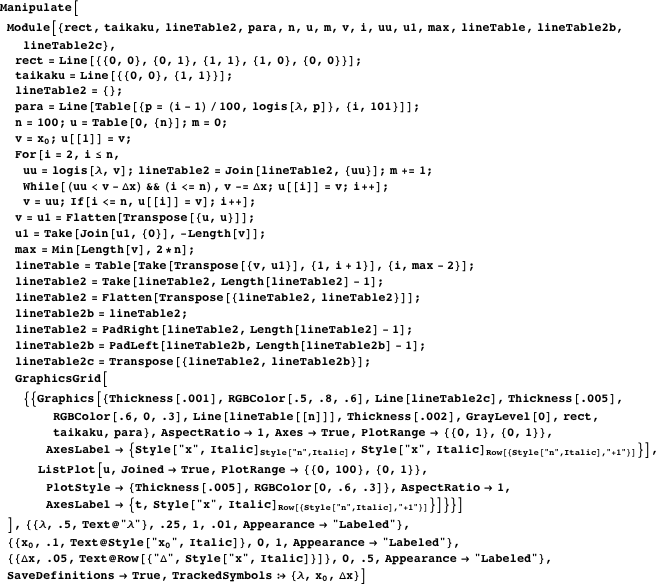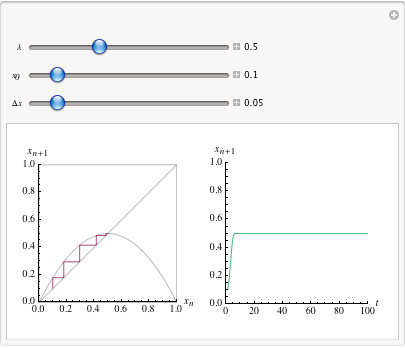Figure 5. Modified logistic map (left) and the generated waveform (right).: the height of the parabola.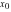: the initial value.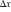: the decreasing speed.

#### 2.5. Examples of Scratching Sound

##### Linearly Modulated Model

The valueis modulated linearly between 0.9 and 0.95.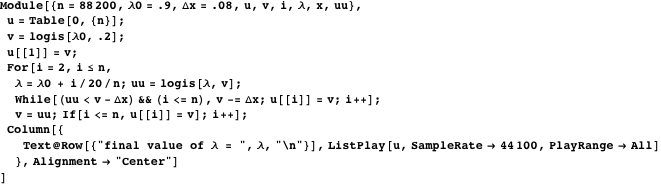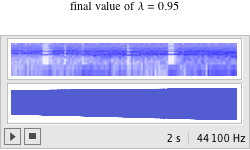Figure 6. Sound generated with the modified logistic model. Sample rate: 44.1 kHz.: linearly driven from 0.9 to 0.95;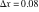.

##### Sinusoidally Modulated Model

The valueis modulated sinusoidally between 0.901 and 0.911.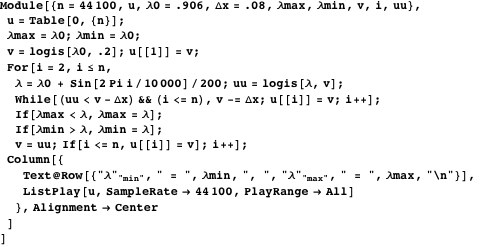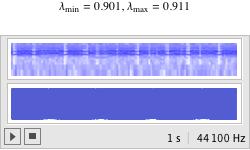Figure 7. Sound generated with the modified logistic model. Sample rate: 44.1 kHz.: sinusoidally driven..

##### Randomly Modulated Model

The valueis modulated with a lowpass-filtered random noise.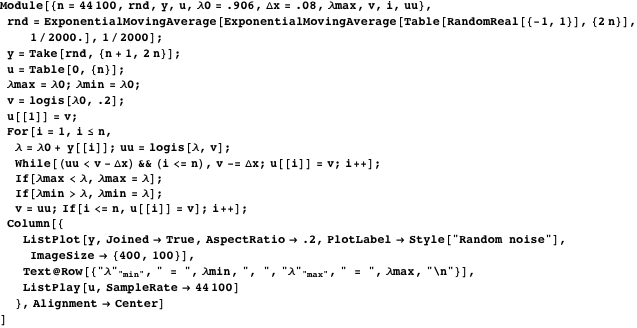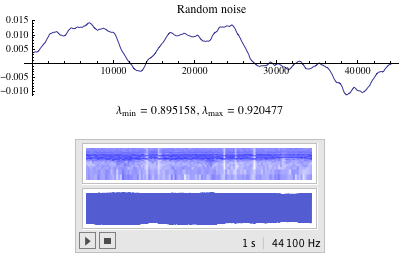Figure 8. Sound generated with the modified logistic model. Sample rate: 44.1 kHz.: randomly driven..

### 3. Discussion

Scratching sounds often produce a strongly uncomfortable sensation, but what causes this feeling remains to be understood. This article does not intend to answer this question, but instead presents models to produce such sounds. As described above, scratching sounds are strongly related to chaos, but chaos in a steady state does not necessarily cause an unpleasant reaction. It might be conjectured that entering or leaving a chaotic domain might be the main factor in this psychoacoustic phenomenon.

Figure 2 from section 2.2 gives the sound generated by the logistic map withvaried from 0.9 to 0.95 at a sample rate of 16 kHz. Figure 9 shows the bifurcation diagram and the corresponding Lyapunov exponents . If the Lyapunov exponent is positive, the system is chaotic; if it is negative, the system becomes stable and converges to a periodically stable state. It is found that stable states appear intermittently in the midst of the chaotic domain.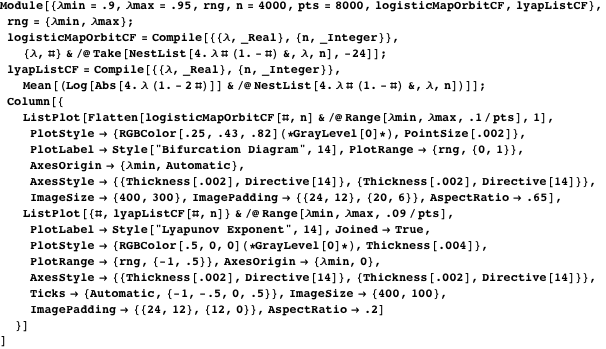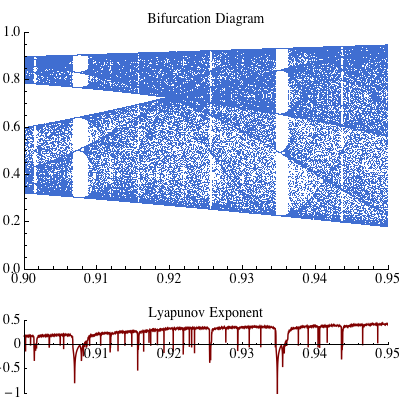Figure 9. The bifurcation diagram and the corresponding Lyapunov exponents .

Figure 10 shows the sound with the valueset to 0.9, which sounds like stationary random noise, and the chilling reaction is less than that of the sound in Figure 2. This result implies that chaos itself does not necessarily cause chills, but rather that chills are due to the transition in and out of chaos.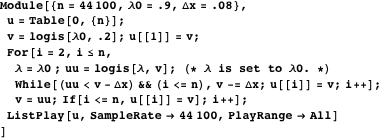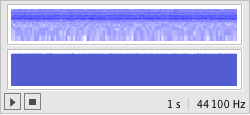Figure 10. Sound generated with the valuefixed to 0.9.

Figure 6 is the sound generated by the proposed stick-slip model withvaried from 0.9 to 0.95 at a sample rate of 44.1 kHz and with the additional parameterset to 0.08. The sound spectrum does not differ much from that of the sound in Figure 2, but this model allows for control of pitch frequency. The sound examples (Figures 6, 7, 8​) are generated with various types of parameter modulations: linear, sinusoidal, and random, respectively. Each modulation range is determined to include at least one Lyapunov dip as shown in Figure 9.

### 4. Summary

It was shown that a mathematical model based on the logistic map produces such sounds as are generated by scratching a chalkboard or glass plate with fingernails. This model exhibits chaotic properties, and unpleasant chilling reactions are found to be strongly related to chaotic behavior, particularly the behavior of entering or leaving chaos. The modified logistic model was presented; it possesses the stick-slip property of the frictional motions. However, a psychoacoustic explanation of reactions to such sounds still remains to be understood.

### References

  T. J. Cox, “Scraping Sounds and Disgusting Noises,” Applied Acoustics, 69(12), 2008 pp. 1195-1204. doi:10.1016/j.apacoust.2007.11.004.  D. L. Halpern, R. Blake, and J. Hillenbrand, “Psychoacoustics of a Chilling Sound,” Perception & Psychophysics 39(2), 1986 pp. 77-80. doi:10.3758/BF03211488.  P. W. Milonni, M-L. Shih, and J. R. Ackerhalt, Chaos in Laser-Matter Interactions, Singapore: World Scientific Publishing, 1987.  Wolfram Research. “Logistic Map.” (Nov 30, 2012) reference.wolfram.com/legacy/v5_2/Demos/SoundGallery/LogisticMap.html.  E. Mahieu. “Lyapunov Exponents for the Logistic Map” from the Wolfram Demonstrations Project—A Wolfram Web Resource. www.demonstrations.wolfram.com/LyapunovExponentsForTheLogisticMap. Y. Miki, “Using the Logistic Map to Generate Scratching Sounds,” The Mathematica Journal, 2013. dx.doi.org/doi:10.3888/tmj.15-5.